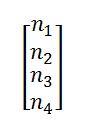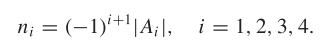# Kernel vector of statics Jacobian

Hi all,

I was reading an article that utilized a 3x4 statics Jacobian and said to calculate the kernel vector:You can row by row, whereWhere Ai is the statics Jacobian with the ith column removed. The problem is I have a 3x3 statics Jacobian, so if I remove the ith column I will end up with a non-square matrix, which means taking the determinant would not be possible. Is there another way to find the kernel vector in a similar way? Could I not remove the column?

Thanks!